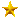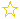营业执照房源信息营业执照房源信息营业执照房源信息营业执照房源信息营业执照房源信息营业执照房源信息营业执照房源信息营业执照房源信息

• 出租
• [三缘商业地产]
• 出租
• [三缘商业地产]
• 出租
• [三缘商业地产]
• 出租
• [三缘商业地产]
• 出租
• [三缘商业地产]
• 出租
• [三缘商业地产]
• 出租
• [三缘商业地产]
• 出租
• [三缘商业地产]
• 出租
• [三缘商业地产]
• 出租
• [三缘商业地产]
• 出租
• [三缘商业地产]
• 出售
• [三缘商业地产]
• 出租
• [三缘商业地产]
• 出租
• [三缘商业地产]
• 出售
• [三缘商业地产]
• 出租
• [三缘商业地产]
• 出售
• [三缘商业地产]
• 出售
• [三缘商业地产]
• 出租
• [三缘商业地产]
• 出售
• [三缘商业地产]
• 出租
• [三缘商业地产]
• 出租
• [三缘商业地产]
• 出租
• [三缘商业地产]
• 出租
• [三缘商业地产]
• 出租
• [三缘商业地产]
• 出租
• [三缘商业地产]
• 出租
• [三缘商业地产]
• 出售
• [振嘉地产]
• 出租
• [振嘉地产]
• 出售
• [振嘉地产]
• 出租
• [振嘉地产]
• 出租
• [振嘉地产]
• 出售
• [振嘉地产]
• 出售
• [振嘉地产]
• 出售
• [振嘉地产]
• 出租
• [振嘉地产]
• 出售
• [振嘉地产]
• 出租
• [振嘉地产]
• 出售
• [振嘉地产]
• 出租
• [振嘉地产]
• 出租
• [振嘉地产]
• 出租
• [振嘉地产]
• 出租
• [振嘉地产]
• 出租
• [振嘉地产]
• 出租
• [振嘉地产]
• 出租
• [轩耀企服]
• 出租
• [轩耀企服]
• 出售
• [嘉腾地产]
• 出售
• [轩耀企服]
• 出租
• [轩耀企服]
• 出租
• [轩耀企服]
• 出售
• [嘉腾地产]
• 出租
• [轩耀企服]
• 出售
• [嘉腾地产]
• 出售
• [左邻右舍]
• 出售
• [嘉腾地产]
• 出租
• [嘉腾地产]
• 出售
• [嘉腾地产]
• 出租
• [左邻右舍]
• 出售
• [嘉腾地产]
• 出售
• [左邻右舍]
• 出售
• [嘉腾地产]
• 出售
• [左邻右舍]
• 出租
• [左邻右舍]
• 出售
• [轩耀企服]
• 出售
• [轩耀企服]
• 出租
• [轩耀企服]
• 出售
• [轩耀企服]
• 出租
• [轩耀企服]
• 出售
• [轩耀企服]
• 出租
• [轩耀企服]
• 出租
• [轩耀企服]
• 出租
• [轩耀企服]
• 出租
• [轩耀企服]
• 出租
• [轩耀企服]
• 出租
• [轩耀企服]
• 出租
• [轩耀企服]
• 出租
• [轩耀企服]
• 出租
• [轩耀企服]
• 出售
• [轩耀企服]
• 出租
• [左邻右舍]
• 出租
• [左邻右舍]
• 出售
• [嘉腾地产]
• 出售
• [嘉腾地产]
• 出售
• [嘉腾地产]
• 出售
• [嘉腾地产]
• 出售
• [嘉腾地产]
• 出售
• [嘉腾地产]
• 出售
• [嘉腾地产]
• 出售
• [嘉腾地产]
• 出售
• [轩耀企服]
• 出租
• [轩耀企服]
• 出租
• [轩耀企服]
• 出租
• [轩耀企服]
• 出租
• [轩耀企服]
• 出租
• [左邻右舍]
• 转让
• [左邻右舍]
• 出租
• [左邻右舍]